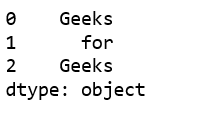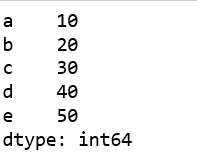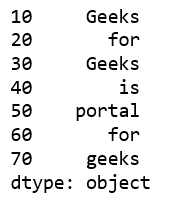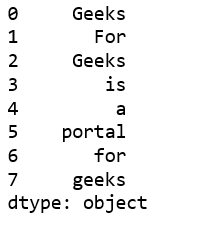Open in App
Not now

# Creating a Pandas Series from Lists

• Difficulty Level : Medium
• Last Updated : 06 Jan, 2019

A `Series` is a one-dimensional labeled array capable of holding any data type (integers, strings, floating point numbers, Python objects, etc.). It has to be remembered that unlike Python lists, a Series will always contain data of the same type.

Let’s see how to create a Pandas Series from lists.

Method #1 : Using `Series()` method without any argument.

 `# import pandas as pd``import` `pandas as pd`` ` `# create Pandas Series with default index values``# default index ranges is from 0 to len(list) - 1``x ``=` `pd.Series([``'Geeks'``, ``'for'``, ``'Geeks'``])`` ` `# print the Series``print``(x)`

Output :Method #2 : Using `Series()` method with `'index'` argument.

 `# import pandas lib. as pd``import` `pandas as pd`` ` `# create Pandas Series with define indexes``x ``=` `pd.Series([``10``, ``20``, ``30``, ``40``, ``50``], index ``=``[``'a'``, ``'b'``, ``'c'``, ``'d'``, ``'e'``])`` ` `# print the Series``print``(x)`

Output :Another example:

 `# import pandas lib. as pd``import` `pandas as pd`` ` `ind ``=` `[``10``, ``20``, ``30``, ``40``, ``50``, ``60``, ``70``]`` ` `lst ``=` `[``'Geeks'``, ``'for'``, ``'Geeks'``, ``'is'``,``            ``'portal'``, ``'for'``, ``'geeks'``]``             ` `# create Pandas Series with define indexes``x ``=` `pd.Series(lst, index ``=` `ind)`` ` `# print the Series``print``(x)`

Output:Method #3: Using `Series()` method with multi-list

 `# importing pandas ``import` `pandas as pd`` ` `# multi-list``list` `=` `[ [``'Geeks'``], [``'For'``], [``'Geeks'``], [``'is'``],``         ``[``'a'``], [``'portal'``], [``'for'``], [``'geeks'``] ]``          ` `# create Pandas Series``df ``=` `pd.Series((i[``0``] ``for` `i ``in` `list``))`` ` `print``(df)`

Output:My Personal Notes arrow_drop_up